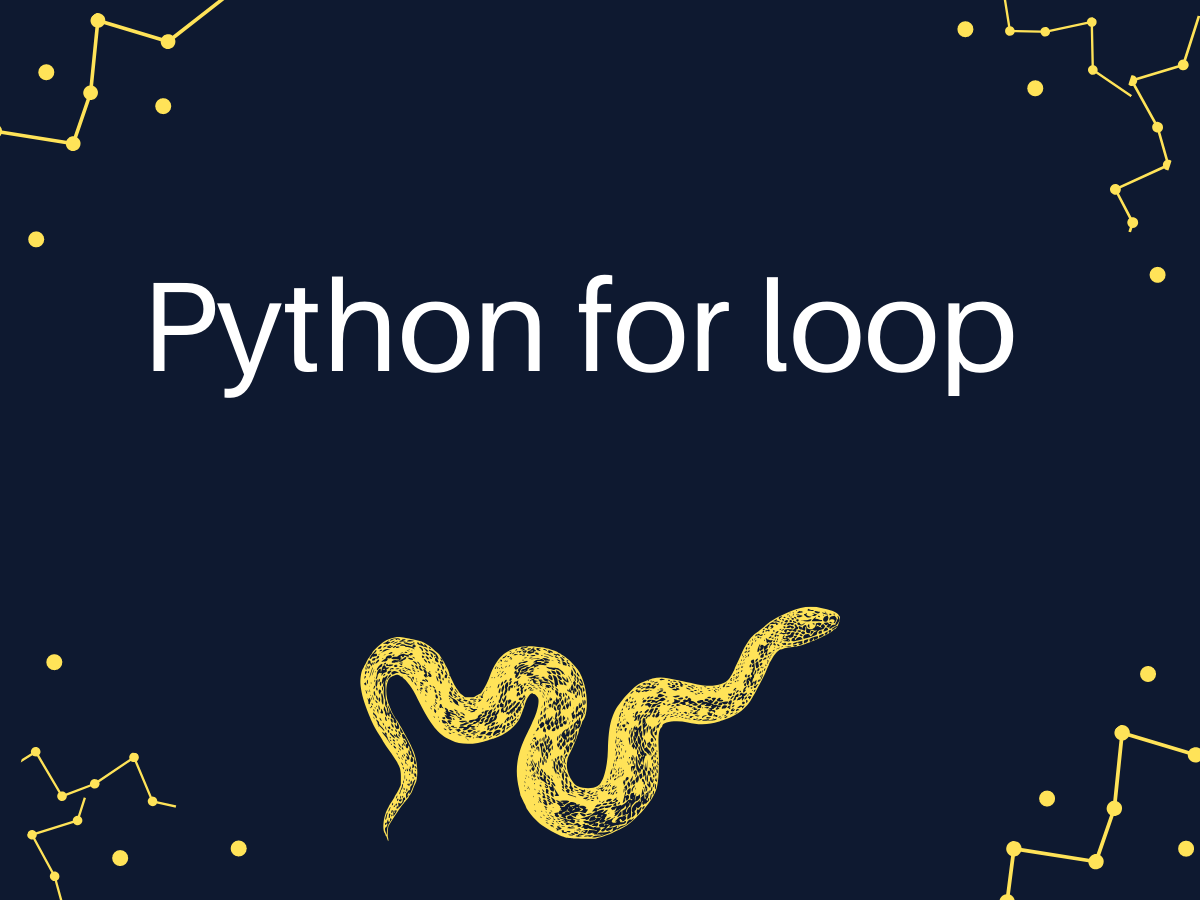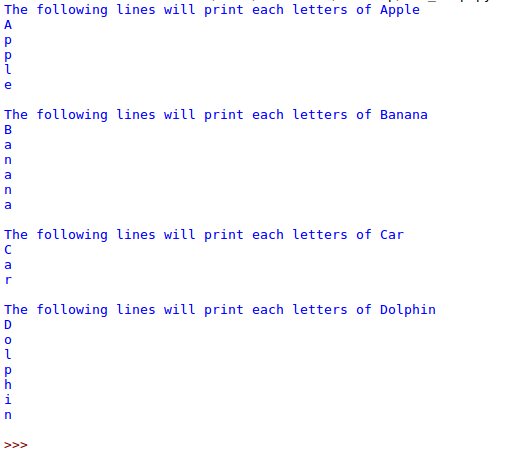# Python for loop [with easy examples]

Published on August 3, 2022By Pankaj
Developer and author at DigitalOcean.While we believe that this content benefits our community, we have not yet thoroughly reviewed it. If you have any suggestions for improvements, please let us know by clicking the “report an issue“ button at the bottom of the tutorial.

The for loop in Python is an iterating function. If you have a sequence object like a list, you can use the for loop to iterate over the items contained within the list.

The functionality of the for loop isn’t very different from what you see in multiple other programming languages.

In this article, we’ll explore the Python for loop in detail and learn to iterate over different sequences including lists, tuples, and more. Additionally, we’ll learn to control the flow of the loop using the break and continue statements.

## Basic Syntax of the Python for loop

The basic syntax of the for loop in Python looks something similar to the one mentioned below.

``````for itarator_variable in sequence_name:
Statements
. . .
Statements

``````

Let me explain the syntax of the Python for loop better.

• The first word of the statement starts with the keyword “for” which signifies the beginning of the for loop.
• Then we have the iterator variable which iterates over the sequence and can be used within the loop to perform various functions
• The next is the “in” keyword in Python which tells the iterator variable to loop for elements within the sequence
• And finally, we have the sequence variable which can either be a list, a tuple, or any other kind of iterator.
• The statements part of the loop is where you can play around with the iterator variable and perform various function

### 1. Print individual letters of a string using the for loop

Python string is a sequence of characters. If within any of your programming applications, you need to go over the characters of a string individually, you can use the for loop here.

Here’s how that would work out for you.

``````word="anaconda"
for letter in word:
print (letter)

``````

Output:

``````a
n
a
c
o
n
d
a
``````

The reason why this loop works is because Python considers a “string” as a sequence of characters instead of looking at the string as a whole.

### 2. Using the for loop to iterate over a Python list or tuple

Lists and Tuples are iterable objects. Let’s look at how we can loop over the elements within these objects now.

``````words= ["Apple", "Banana", "Car", "Dolphin" ]
for word in words:
print (word)
``````

Output:

``````Apple
Banana
Car
Dolphin

``````

Now, let’s move ahead and work on looping over the elements of a tuple here.

``````nums = (1, 2, 3, 4)

sum_nums = 0

for num in nums:
sum_nums = sum_nums + num

print(f'Sum of numbers is {sum_nums}')

# Output
# Sum of numbers is 10

``````

### 3. Nesting Python for loops

When we have a for loop inside another for loop, it’s called a nested for loop. There are multiple applications of a nested for loop.

Consider the list example above. The for loop prints out individual words from the list. But what if we want to print out the individual characters of each of the words within the list instead?

This is where a nested for loop works better. The first loop (parent loop) will go over the words one by one. The second loop (child loop) will loop over the characters of each of the words.

``````words= ["Apple", "Banana", "Car", "Dolphin" ]
for word in words:
#This loop is fetching word from the list
print ("The following lines will print each letters of "+word)
for letter in word:
#This loop is fetching letter for the word
print (letter)
print("") #This print is used to print a blank line

``````

Output### 4. Python for loop with range() function

Python range() is one of the built-in functions. When you want the for loop to run for a specific number of times, or you need to specify a range of objects to print out, the range function works really well. Consider the following example where I want to print the numbers 1, 2, and 3.

``````for x in range(3):
print("Printing:", x)

# Output

# Printing: 0
# Printing: 1
# Printing: 2

``````

The range function also takes another parameter apart from the start and the stop. This is the step parameter. It tells the range function how many numbers to skip between each count.

In the below example, I’ve used number 3 as the step and you can see the output numbers are the previous number + 3.

``````for n in range(1, 10, 3):
print("Printing with step:", n)

# Output

# Printing with step: 1
# Printing with step: 4
# Printing with step: 7

``````

### 5. break statement with for loop

The break statement is used to exit the for loop prematurely. It’s used to break the for loop when a specific condition is met.

Let’s say we have a list of numbers and we want to check if a number is present or not. We can iterate over the list of numbers and if the number is found, break out of the loop because we don’t need to keep iterating over the remaining elements.

In this case, we’ll use the Python if else condition along with our for loop.

``````nums = [1, 2, 3, 4, 5, 6]

n = 2

found = False
for num in nums:
if n == num:
found = True
break

print(f'List contains {n}: {found}')

# Output
# List contains 2: True

``````

### 6. The continue statement with for loop

We can use continue statements inside a for loop to skip the execution of the for loop body for a specific condition.

Let’s say we have a list of numbers and we want to print the sum of positive numbers. We can use the continue statements to skip the for loop for negative numbers.

``````nums = [1, 2, -3, 4, -5, 6]

sum_positives = 0

for num in nums:
if num < 0:
continue
sum_positives += num

print(f'Sum of Positive Numbers: {sum_positives}')

``````

### 6. Python for loop with an else block

We can use else block with a Python for loop. The else block is executed only when the for loop is not terminated by a break statement.

Let’s say we have a function to print the sum of numbers if and only if all the numbers are even.

We can use break statement to terminate the for loop if an odd number is present. We can print the sum in the else part so that it gets printed only when the for loop is executed normally.

``````def print_sum_even_nums(even_nums):
total = 0

for x in even_nums:
if x % 2 != 0:
break

total += x
else:
print("For loop executed normally")
print(f'Sum of numbers {total}')

# this will print the sum
print_sum_even_nums([2, 4, 6, 8])

# this won't print the sum because of an odd number in the sequence
print_sum_even_nums([2, 4, 5, 8])

# Output

# For loop executed normally
# Sum of numbers 20

``````

## Conclusion

The for loop in Python is very similar to other programming languages. We can use break and continue statements with for loop to alter the execution. However, in Python, we can have optional else block in for loop too.

I hope you have gained some interesting ideas from the tutorial above. If you have any questions, let us know in the comments below.

Thanks for learning with the DigitalOcean Community. Check out our offerings for compute, storage, networking, and managed databases.Pankaj

author

Developer and author at DigitalOcean.

#### Still looking for an answer?

Ask a questionSearch for more help

JournalDev
DigitalOcean EmployeeSeptember 15, 2020

I keep getting an invalid syntax error when trying to run the code for the Python for use with optional else block. It is saying the inner first bracket is the invalid syntax error- def print_sum_even_nums ([2, 4, 6, 8]):

- Lee

JournalDev
DigitalOcean EmployeeAugust 19, 2020

How to use for loop in calculator program in python using functions optimized code

- G N Deepak Sravan

JournalDev
DigitalOcean EmployeeNovember 22, 2019

Thanks for this lesson. I’ve learned a lot.

- Camel

JournalDev
DigitalOcean EmployeeJune 2, 2018

Hi Pankaj, problem lies with this line “print letter”. It should be print (letter)

JournalDev
DigitalOcean EmployeeJune 2, 2018

Thank you Imtiaz Abedin for this very useful tutorial series on python.

JournalDev
DigitalOcean EmployeeMay 30, 2018

Python tutorials are really very helpful. But there are some errors in codes.Like in python nested loop example in print function.

- Saurabh Suthar